Time: 3 Hours                                                                                                     Max. Marks: 100

NOTE: There are 9 Questions in all.

·      Question 1 is compulsory and carries 20 marks. Answer to Q. 1. must be written in the space provided for it in the answer book supplied and nowhere else.

·      Out of the remaining EIGHT Questions answer any FIVE Questions. Each question carries 16 marks.

·      Any required data not explicitly given, may be suitably assumed and stated.

Q.1       Choose the correct or best alternative in the following:                                         (2x10)

a.       Thermal noise power is proportional to

(A)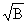.                                            (B)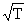.

(C)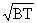.                                         (D)  BT.

b.      If the amplitude of carrier wave is 10 V and the modulation index is 30%, the amplitude of sidebands will be

(A)     1.5 V.                                         (B)  3 V.

(C)  6 V.                                             (D)  30 V.

(A)    15 kHz.                                        (B)  75 kHz.

(C)  100 kHz.                                      (D)  200 kHz.

d.   Pre-emphasis is used to improve noise immunity

(A)    at higher baseband frequencies.

(B)    at lower baseband frequencies.

(C)  over the entire baseband.

(D)  for AM.

e.   VSWR on transmission line lies in the range

(A)     0 to 1.                                          (B)  -1 to +1.

(C)  1 to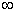.                                        (D)  0 to.

f.    In TV transmission systems, sync-pulses are accommodated during

(A)     colour burst.                                 (B)  horizontal blanking.

(C)  vertical blanking.                           (D)  none of these.

g.   The dominant mode in a rectangular waveguide is

(A)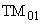.                                        (B)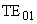.

(C)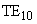.                                          (D)  TEM.

h.   The quantization error in a PCM system depends on the

(A)    sampling rate.                               (B)  number of quantization levels.

(C) signal bandwidth.                           (D) shape of the pulses.

i.    The signals sent by the TV transmitter to ensure correct scanning in the receiver are called

(A)   sync.                                            (B) chroma.

(C) luminance.                                     (D) video.

j.    Thermal noise in communication system is

(A)  internal and continuous.                 (B)  internal and impulsive.

(C)  external and continuous.                (D)  external and impulsive.

Answer any FIVE Questions out of EIGHT Questions.

Each question carries 16 marks.

Q.2     a.   Discuss a method of generating SSB signals.                                                       (8)

b.   Derive the relation between noise figure and noise temperature of a system.         (8)

Q.3     a.   Explain amplitude modulation. Derive the relation between the output power of an AM transmitter and the depth of modulation.                            (8)

b.   A transmitter with 10 kW carrier transmits 11.2 kW when modulated with a single sine wave.  Calculate the modulation index.  If the carrier is simultaneously modulated with another sine wave at 50% modulation, find the total transmitted power.                                                                               (8)

Q.4     a.   Draw the block diagram of a superheterodyne radio receiver and explain its working.                     (8)

b.   Explain, with a circuit diagram, the working of a slope detector.                          (8)

Q.5     a.   What is pulse code modulation?  Explain with the help of a block diagram.          (8)

b.   What is frequency modulation?  Derive an expression for frequency-modulated wave.                     (8)

Q.6     a.   Explain how an FM signal can be demodulated using a PLL.                               (8)

b.   What are the different types of losses in optical fibers?  Explain.                          (8)

Q.7     a.   Explain how the dominant mode is generated in a rectangular waveguide.            (8)

b.   Write  a note on pulse position modulation.                                                         (8)

Q.8     a.   Explain the following terms in relation to the ionosphere:

(i)                  Virtual height.

(ii)                MUF.                                                                                        (8)

b.   Describe the operation of a rhombic antenna.                                                      (8)

Q.9     a.   Describe briefly the use of blanking and synchronizing pulses in TV signals.                         (8)